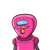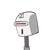# I. Fill in the blanks: 1. The place value of 4 in 26,74,19,985 is __________. 2. The short form of 9,00,00,000 + 2,00

I. Fill in the blanks:
1. The place value of 4 in 26,74,19,985 is __________.
2. The short form of 9,00,00,000 + 2,00,000 + 8,000 + 500 + 20 + 7 is __________.
3. Put the sign ( >, <, or =) in the blanks: -15 __________ +23
4. Multiply: 8438.06 x 100 = __________
5. The absolute value of -8 is __________.
6. Simplify: -3 + (+5) __________.
II. Do as directed:
1. Write in words using Indian place value system: 75,30,04,815
2. Write in figures: Eight hundred fifteen million, nine hundred three thousand,
four hundred six.
3. Write in expanded form: 37,58,60,142
4. Write the greatest and the smallest 4-digit number without repeating a digit from
the following digits: 2, 8, 9, 6
5. Arrange the following integers in increasing (ascending) order:
-15, +4, -9, +8, 0, -12, -17, +12
6. Convert 15 hectometer into decimeter.
7. Convert 4,319 milliliter into liter.
8. Arrange in columns and add: 3,21,35,073 + 1,92,73,985 + 85,37,926 
9. Find the difference between 1,93,56,241 and 18,65,407
10.Divide: 63,54,192 ÷ 15
11.Add: 72.423 ; 28.94 ; and 43.762
12.Multiply: 8.23 x 5.6
13.Subtract 75 km 843 m from 106 km 250 m
1. Find the area of square, whose side is 20 m.
2. Find the area of rectangle, whose length 12 cm and breadth 10 cm.

### 2 thoughts on “I. Fill in the blanks: <br /> 1. The place value of 4 in 26,74,19,985 is __________.<br /> 2. The short form of 9,00,00,000 + 2,00”

1.1. 4,00,000

2. 90208527

3. <

4. 843806

5. 8

6. 2

1. Area of sq is. a2

= 20×20

= 400 m2

2. Area of rectangle = l × b

= 12× 10

= 120 m2

2.